Скачать презентацию PH 201 Dr Cecilia Vogel Lecture 2

0f6e0ebdf2a2e9bab6073e683378bc66.ppt

• Количество слайдов: 20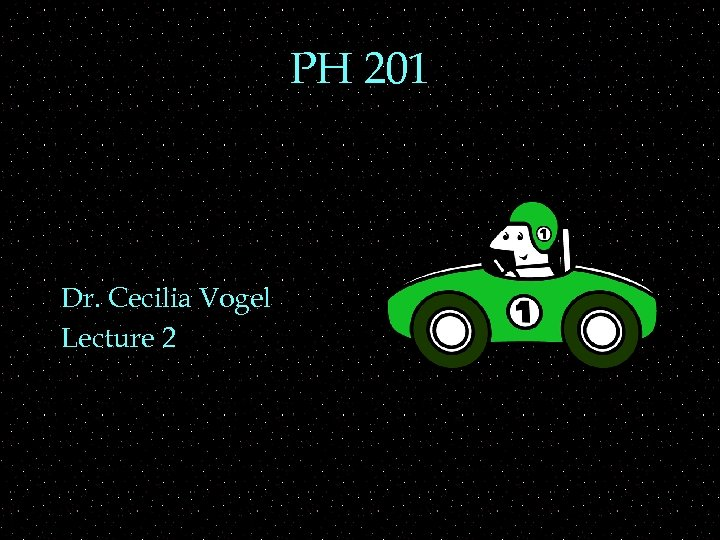PH 201 Dr. Cecilia Vogel Lecture 2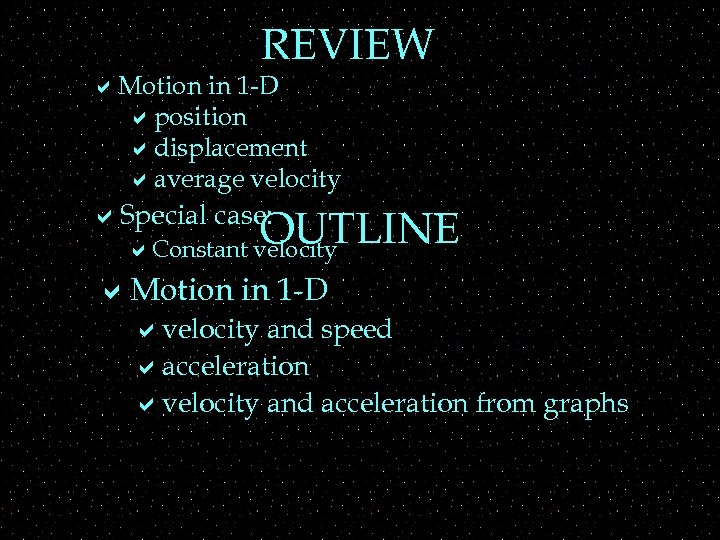REVIEW a. Motion in 1 -D aposition adisplacement aaverage velocity a. Special case: OUTLINE a. Constant velocity a. Motion in 1 -D avelocity and speed aacceleration avelocity and acceleration from graphs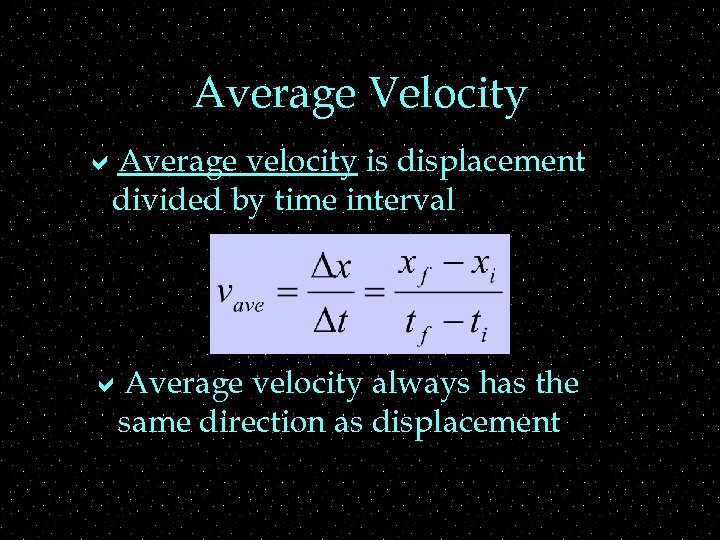Average Velocity a. Average velocity is displacement divided by time interval a. Average velocity always has the same direction as displacement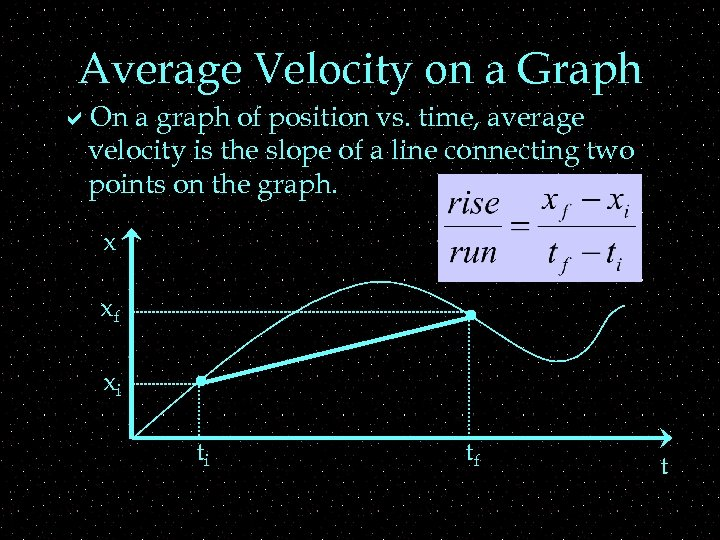Average Velocity on a Graph a. On a graph of position vs. time, average velocity is the slope of a line connecting two points on the graph. x xf xi ti tf t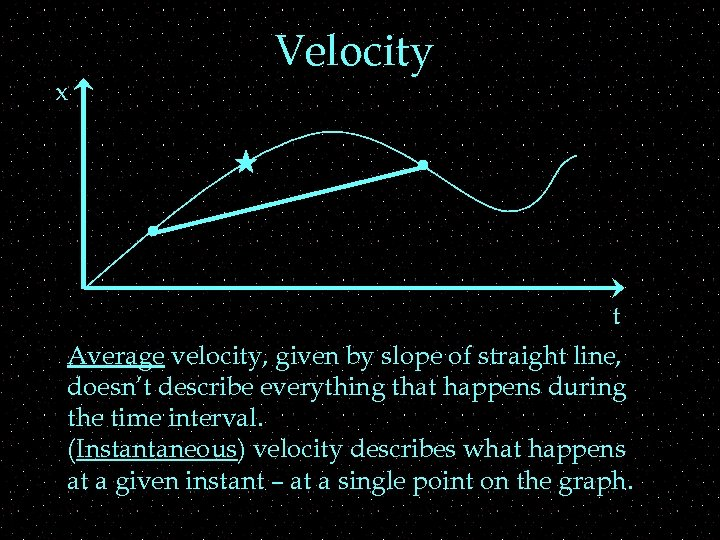x Velocity t Average velocity, given by slope of straight line, doesn’t describe everything that happens during the time interval. (Instantaneous) velocity describes what happens at a given instant – at a single point on the graph.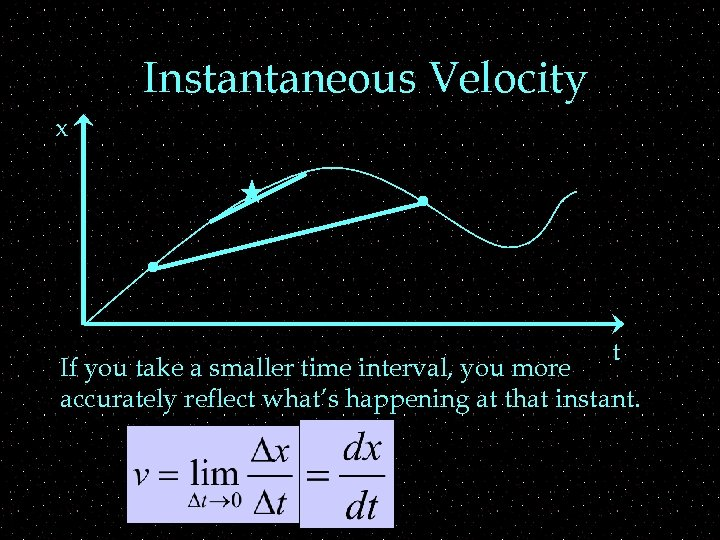Instantaneous Velocity x t If you take a smaller time interval, you more accurately reflect what’s happening at that instant.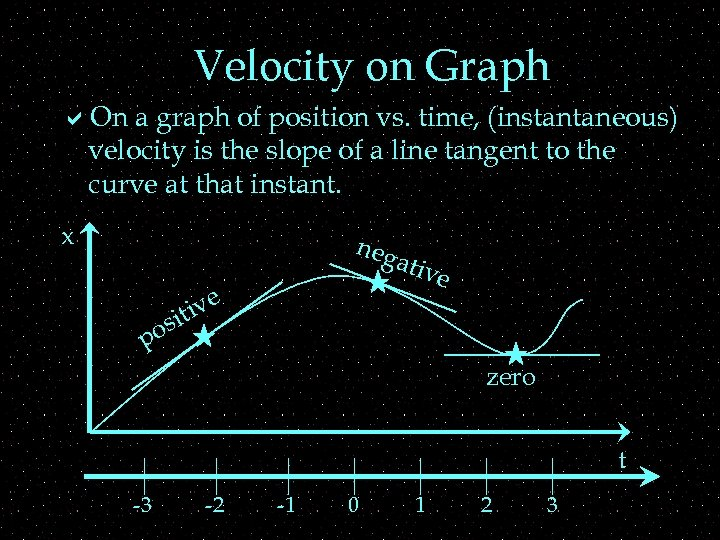Velocity on Graph a. On a graph of position vs. time, (instantaneous) velocity is the slope of a line tangent to the curve at that instant. x s po neg ativ e ive it zero t -3 -2 -1 0 1 2 3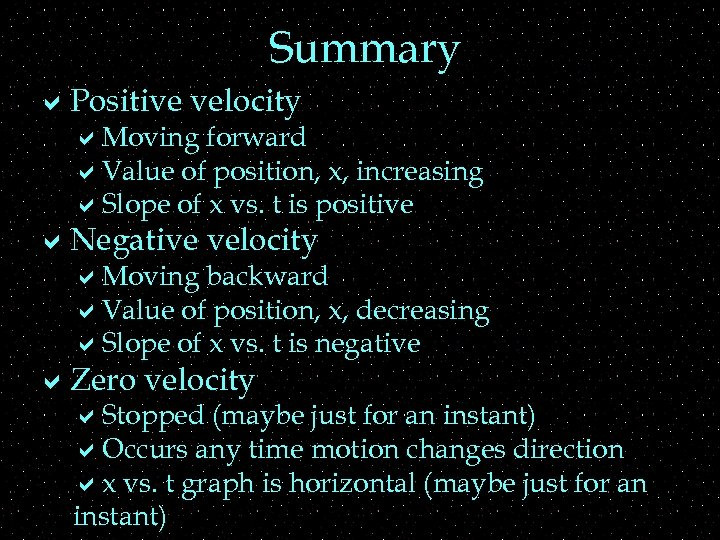Summary a. Positive velocity a. Moving forward a. Value of position, x, increasing a. Slope of x vs. t is positive a. Negative velocity a. Moving backward a. Value of position, x, decreasing a. Slope of x vs. t is negative a. Zero velocity a. Stopped (maybe just for an instant) a. Occurs any time motion changes direction ax vs. t graph is horizontal (maybe just for an instant)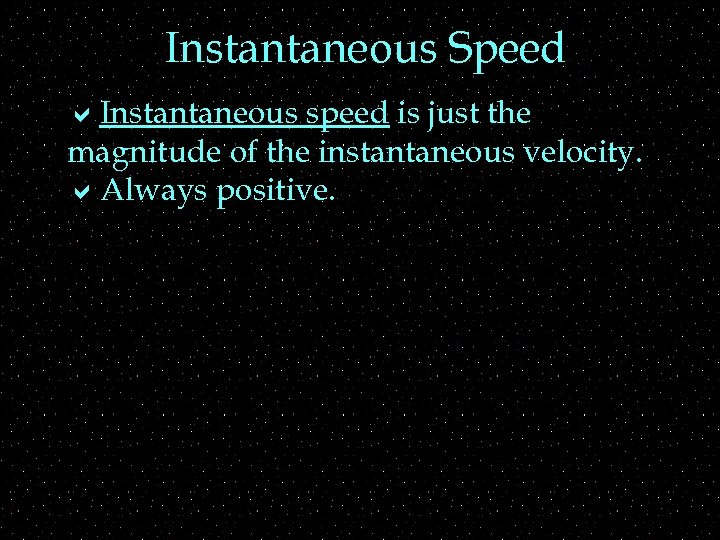Instantaneous Speed a. Instantaneous speed is just the magnitude of the instantaneous velocity. a. Always positive.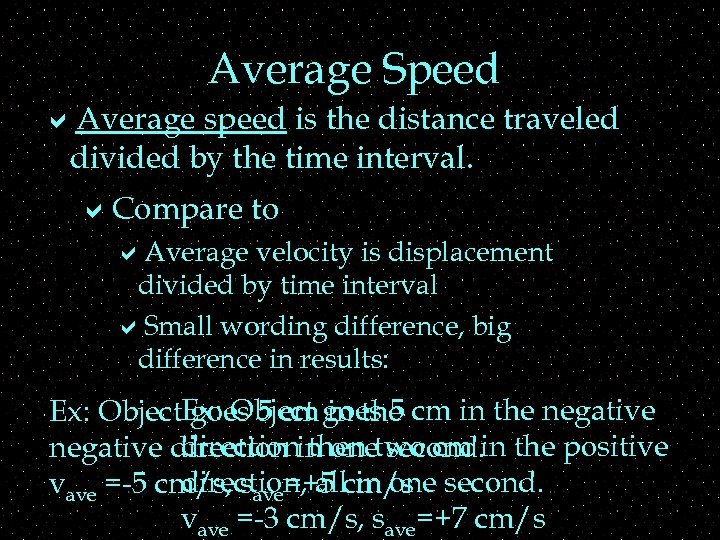Average Speed a. Average speed is the distance traveled divided by the time interval. a. Compare to a. Average velocity is displacement divided by time interval a. Small wording difference, big difference in results: Ex: Object goes 5 cm in the negative goes 5 cm in the direction then second. negative direction in onetwo cm in the positive direction, all in one vave =-5 cm/s, save=+5 cm/s second. vave =-3 cm/s, save=+7 cm/s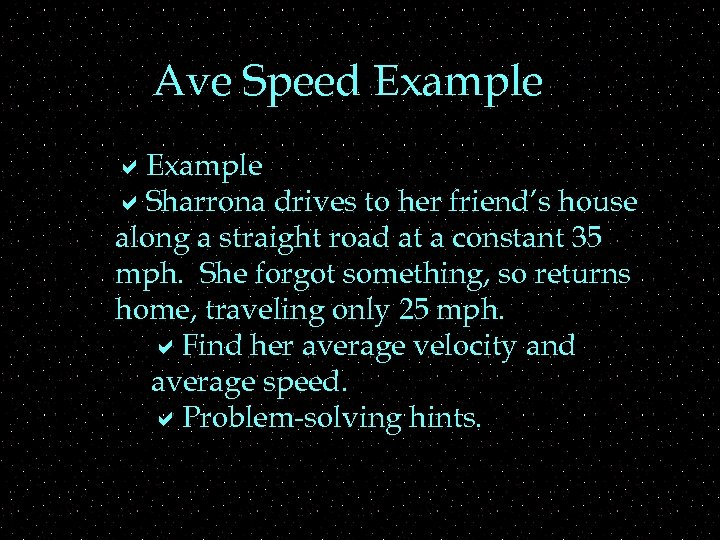Ave Speed Example a. Sharrona drives to her friend’s house along a straight road at a constant 35 mph. She forgot something, so returns home, traveling only 25 mph. a. Find her average velocity and average speed. a. Problem-solving hints.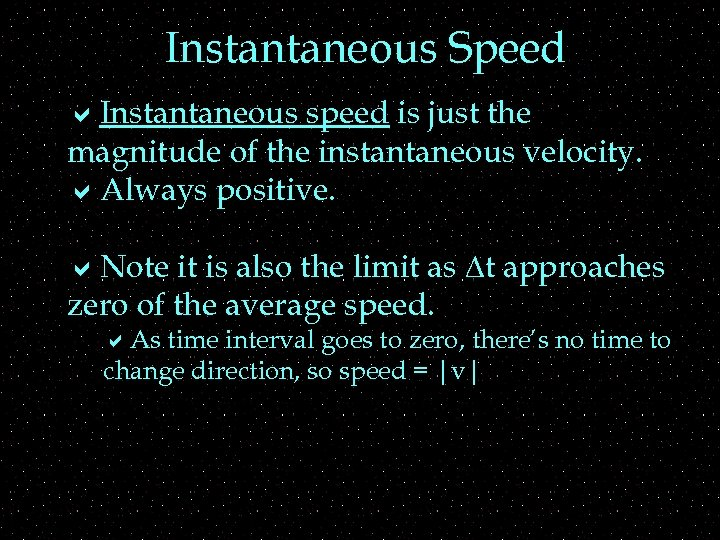Instantaneous Speed a. Instantaneous speed is just the magnitude of the instantaneous velocity. a. Always positive. a. Note it is also the limit as Dt approaches zero of the average speed. a. As time interval goes to zero, there’s no time to change direction, so speed = |v|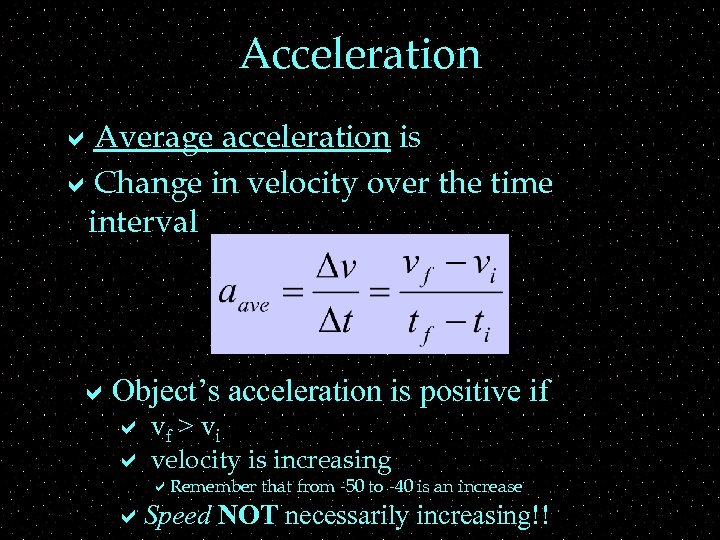Acceleration a. Average acceleration is a. Change in velocity over the time interval a. Object’s acceleration is positive if a vf > vi a velocity is increasing a. Remember that from -50 to -40 is an increase a. Speed NOT necessarily increasing!!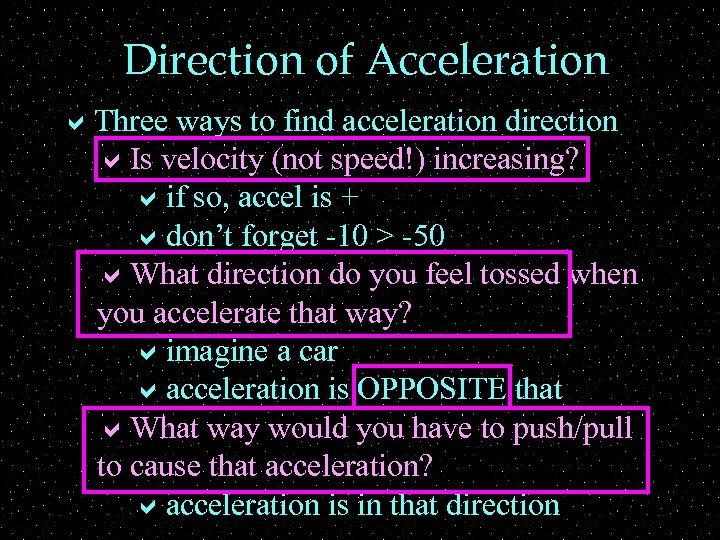Direction of Acceleration a. Three ways to find acceleration direction a. Is velocity (not speed!) increasing? aif so, accel is + adon’t forget -10 > -50 a. What direction do you feel tossed when you accelerate that way? aimagine a car aacceleration is OPPOSITE that a. What way would you have to push/pull to cause that acceleration? aacceleration is in that direction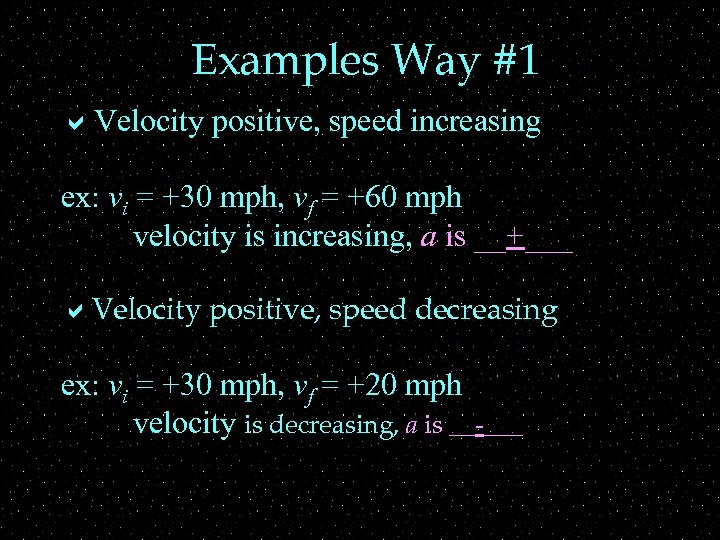Examples Way #1 a. Velocity positive, speed increasing ex: vi = +30 mph, vf = +60 mph velocity is increasing, a is __+___ a. Velocity positive, speed decreasing ex: vi = +30 mph, vf = +20 mph velocity is decreasing, a is __-___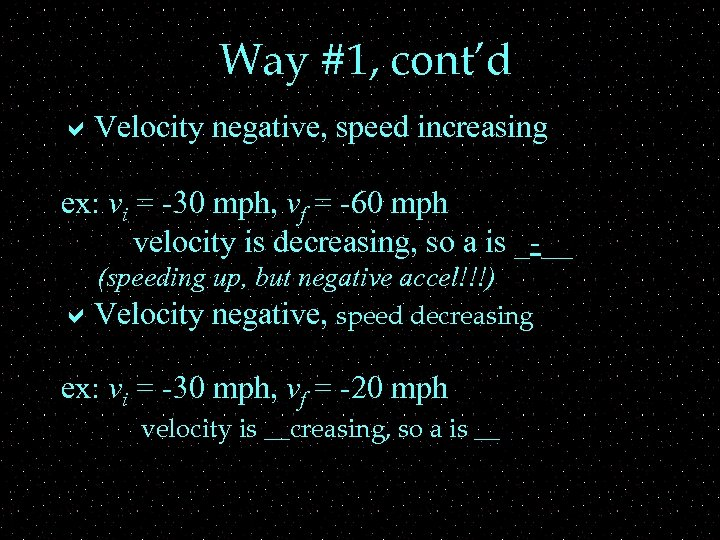Way #1, cont’d a. Velocity negative, speed increasing ex: vi = -30 mph, vf = -60 mph velocity is decreasing, so a is _-__ (speeding up, but negative accel!!!) a. Velocity negative, speed decreasing ex: vi = -30 mph, vf = -20 mph velocity is __creasing, so a is __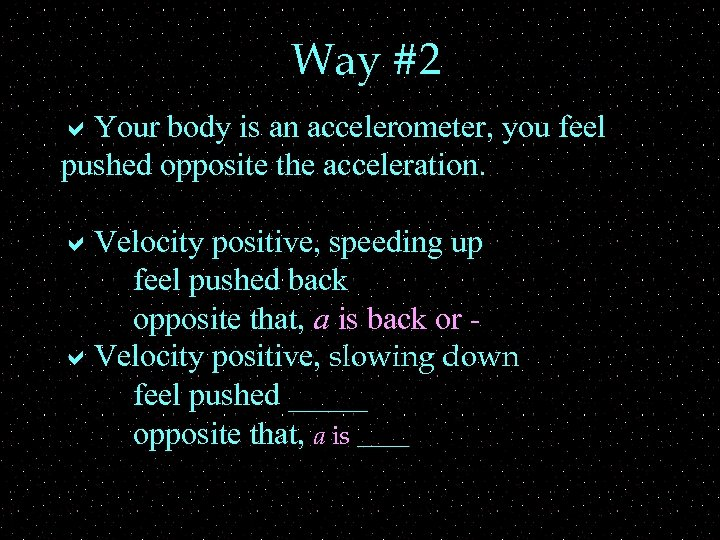Way #2 a. Your body is an accelerometer, you feel pushed opposite the acceleration. a. Velocity positive, speeding up feel pushed back opposite that, a is back or a. Velocity positive, slowing down feel pushed _____ opposite that, a is ____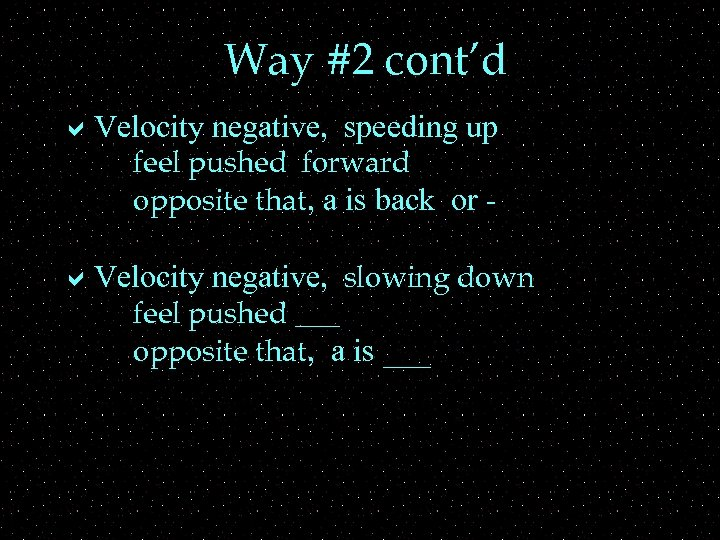Way #2 cont’d a. Velocity negative, speeding up feel pushed forward opposite that, a is back or - a. Velocity negative, slowing down feel pushed ___ opposite that, a is ___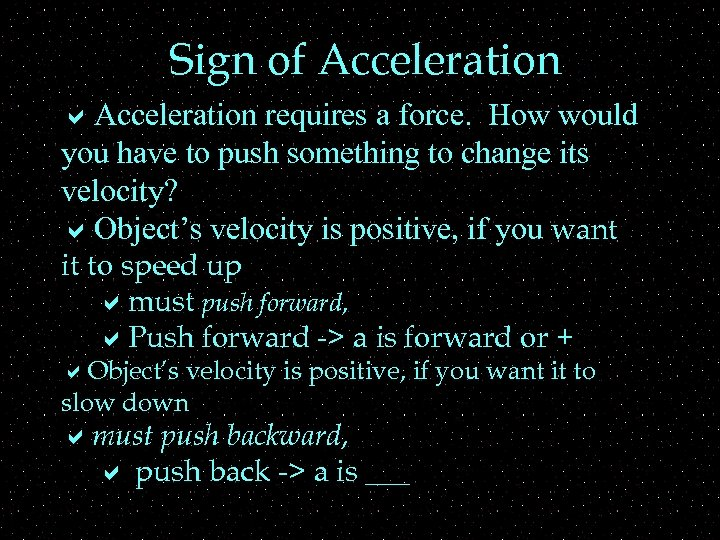Sign of Acceleration a. Acceleration requires a force. How would you have to push something to change its velocity? a. Object’s velocity is positive, if you want it to speed up amust push forward, a. Push forward -> a is forward or + a. Object’s velocity is positive, if you want it to slow down amust push backward, a push back -> a is ___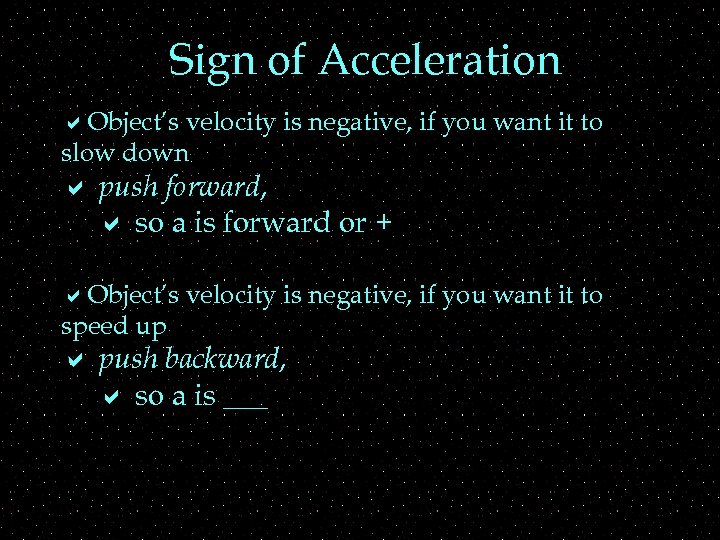Sign of Acceleration a. Object’s velocity is negative, if you want it to slow down a push forward, a so a is forward or + a. Object’s velocity is negative, if you want it to speed up a push backward, a so a is ___